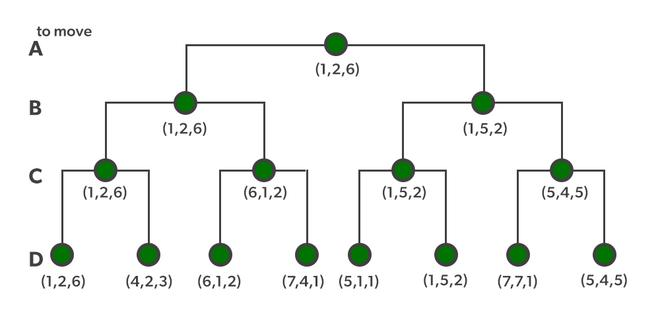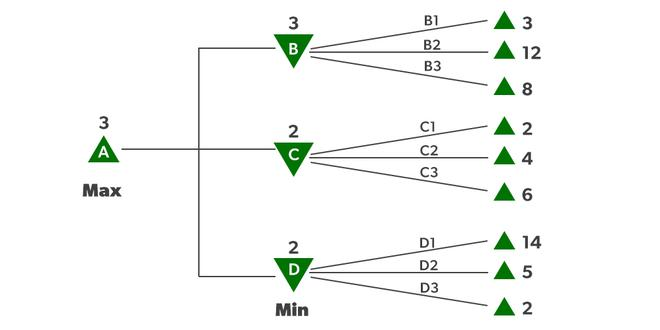# Optimal Decision Making in Games

• Last Updated : 28 Feb, 2022

Humans’ intellectual capacities have been engaged by games for as long as civilization has existed, sometimes to an alarming degree. Games are an intriguing subject for AI researchers because of their abstract character. A game’s state is simple to depict, and actors are usually limited to a small number of actions with predetermined results. Physical games, such as croquet and ice hockey, contain significantly more intricate descriptions, a much wider variety of possible actions, and rather ambiguous regulations defining the legality of activities. With the exception of robot soccer, these physical games have not piqued the AI community’s interest.

Games are usually intriguing because they are difficult to solve. Chess, for example, has an average branching factor of around 35, and games frequently stretch to 50 moves per player, therefore the search tree has roughly 35100 or 10154 nodes (despite the search graph having “only” about 1040 unique nodes). As a result, games, like the real world, necessitate the ability to make some sort of decision even when calculating the best option is impossible.

Inefficiency is also heavily punished in games. Whereas a half-efficient implementation of A search will merely take twice as long to complete, a chess software that is half as efficient in utilizing its available time will almost certainly be beaten to death, all other factors being equal. As a result of this research, a number of intriguing suggestions for making the most use of time have emerged.

### Optimal Decision Making in Games

Let us start with games with two players, whom we’ll refer to as MAX and MIN for obvious reasons. MAX is the first to move, and then they take turns until the game is finished. At the conclusion of the game, the victorious player receives points, while the loser receives penalties. A game can be formalized as a type of search problem that has the following elements:

• S0: The initial state of the game, which describes how it is set up at the start.
• Player (s): Defines which player in a state has the move.
• Actions (s): Returns a state’s set of legal moves.
• Result (s, a): A transition model that defines a move’s outcome.
• Terminal-Test (s): A terminal test that returns true if the game is over but false otherwise. Terminal states are those in which the game has come to a conclusion.
• Utility (s, p): A utility function (also known as a payout function or objective function ) determines the final numeric value for a game that concludes in the terminal state s for player p. The result in chess is a win, a loss, or a draw, with values of +1, 0, or 1/2. Backgammon’s payoffs range from 0 to +192, but certain games have a greater range of possible outcomes. A zero-sum game is defined (confusingly) as one in which the total reward to all players is the same for each game instance. Chess is a zero-sum game because each game has a payoff of 0 + 1, 1 + 0, or 1/2 + 1/2. “Constant-sum” would have been a preferable name, 22 but zero-sum is the usual term and makes sense if each participant is charged 1.

The game tree for the game is defined by the beginning state, ACTIONS function, and RESULT function—a tree in which the nodes are game states and the edges represent movements. The figure below depicts a portion of the tic-tac-toe game tree (noughts and crosses). MAX may make nine different maneuvers from his starting position. The game alternates between MAXs setting an X and MINs placing an O until we reach leaf nodes corresponding to terminal states, such as one player having three in a row or all of the squares being filled. The utility value of the terminal state from the perspective of MAX is shown by the number on each leaf node; high values are thought to be beneficial for MAX and bad for MIN

The game tree for tic-tac-toe is relatively short, with just 9! = 362,880 terminal nodes. However, because there are over 1040 nodes in chess, the game tree is better viewed as a theoretical construct that cannot be realized in the actual world. But, no matter how big the game tree is, MAX’s goal is to find a solid move. A tree that is superimposed on the whole game tree and examines enough nodes to allow a player to identify what move to make is referred to as a search tree.A sequence of actions leading to a goal state—a terminal state that is a win—would be the best solution in a typical search problem. MIN has something to say about it in an adversarial search. MAX must therefore devise a contingent strategy that specifies M A X’s initial state move, then MAX’s movements in the states resulting from every conceivable MIN response, then MAX’s moves in the states resulting from every possible MIN reaction to those moves, and so on. This is quite similar to the AND-OR search method, with MAX acting as OR and MIN acting as AND. When playing an infallible opponent, an optimal strategy produces results that are as least as excellent as any other plan. We’ll start by demonstrating how to find the best plan.

We’ll move to the trivial game in the figure below since even a simple game like tic-tac-toe is too complex for us to draw the full game tree on one page. MAX’s root node moves are designated by the letters a1, a2, and a3. MIN’s probable answers to a1 are b1, b2, b3, and so on. This game is over after MAX and MIN each make one move. (In game terms, this tree consists of two half-moves and is one move deep, each of which is referred to as a ply.) The terminal states in this game have utility values ranging from 2 to 14.

### Game’s Utility Function

The optimal strategy can be found from the minimax value of each node, which we express as MINIMAX, given a game tree (n). Assuming that both players play optimally from there through the finish of the game, the utility (for MAX) of being in the corresponding state is the node’s minimax value. The usefulness of a terminal state is obviously its minimax value. Furthermore, if given the option, MAX prefers to shift to a maximum value state, whereas MIN wants to move to a minimum value state. So here’s what we’ve got:Optimal Decision Making in Multiplayer Games

Let’s use these definitions to analyze the game tree shown in the figure above. The game’s UTILITY function provides utility values to the terminal nodes on the bottom level. Because the first MIN node, B, has three successor states with values of 3, 12, and 8, its minimax value is 3. Minimax value 2 is also used by the other two MIN nodes. The root node is a MAX node, with minimax values of 3, 2, and 2, resulting in a minimax value of 3. We can also find the root of the minimax decision: action a1 is the best option for MAX since it leads to the highest minimax value.

This concept of optimal MAX play requires that MIN plays optimally as well—it maximizes MAX’s worst-case outcome. What happens if MIN isn’t performing at its best? Then it’s a simple matter of demonstrating that MAX can perform even better. Other strategies may outperform the minimax method against suboptimal opponents, but they will always outperform optimal opponents.

My Personal Notes arrow_drop_up